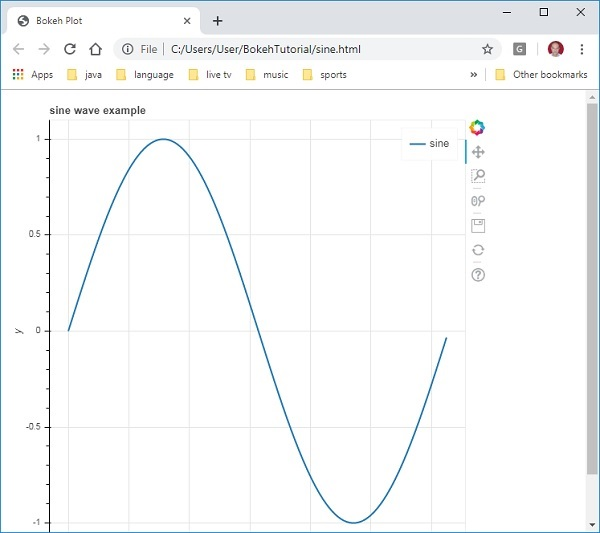# Bokeh - Getting Started

Creating a simple line plot between two numpy arrays is very simple. To begin with, import following functions from bokeh.plotting modules −

```from bokeh.plotting import figure, output_file, show
```

The figure() function creates a new figure for plotting.

The output_file() function is used to specify a HTML file to store output.

The show() function displays the Bokeh figure in browser on in notebook.

Next, set up two numpy arrays where second array is sine value of first.

```import numpy as np
import math
x = np.arange(0, math.pi*2, 0.05)
y = np.sin(x)
```

To obtain a Bokeh Figure object, specify the title and x and y axis labels as below −

```p = figure(title = "sine wave example", x_axis_label = 'x', y_axis_label = 'y')
```

The Figure object contains a line() method that adds a line glyph to the figure. It needs data series for x and y axes.

```p.line(x, y, legend = "sine", line_width = 2)
```

Finally, set the output file and call show() function.

```output_file("sine.html")
show(p)
```

This will render the line plot in ‘sine.html’ and will be displayed in browser.

### Complete code and its output is as follows

```from bokeh.plotting import figure, output_file, show
import numpy as np
import math
x = np.arange(0, math.pi*2, 0.05)
y = np.sin(x)
output_file("sine.html")
p = figure(title = "sine wave example", x_axis_label = 'x', y_axis_label = 'y')
p.line(x, y, legend = "sine", line_width = 2)
show(p)
```

### Output on browser